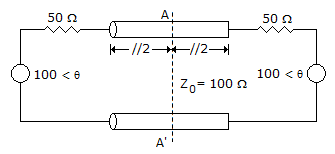Courses

# Test: Electromagnetic Field Theory - 5

## 25 Questions MCQ Test Electromagnetic Theory | Test: Electromagnetic Field Theory - 5

Description
This mock test of Test: Electromagnetic Field Theory - 5 for Electrical Engineering (EE) helps you for every Electrical Engineering (EE) entrance exam. This contains 25 Multiple Choice Questions for Electrical Engineering (EE) Test: Electromagnetic Field Theory - 5 (mcq) to study with solutions a complete question bank. The solved questions answers in this Test: Electromagnetic Field Theory - 5 quiz give you a good mix of easy questions and tough questions. Electrical Engineering (EE) students definitely take this Test: Electromagnetic Field Theory - 5 exercise for a better result in the exam. You can find other Test: Electromagnetic Field Theory - 5 extra questions, long questions & short questions for Electrical Engineering (EE) on EduRev as well by searching above.
QUESTION: 1

Solution:
QUESTION: 2

Solution:
QUESTION: 3

### The attenuation in a waveguide near the cut off frequency is

Solution:
QUESTION: 4

The electric flux and field intensity inside a conducting sphere is

Solution:
QUESTION: 5

T.V. antennas are usually

Solution:
QUESTION: 6

Measurement of radiation resistance by reflection method is suitable for

Solution:
QUESTION: 7

A lossless transmission line is connected to two identical voltage source as shown in figure.The impedance measured at the midpoint AA' is

Solution:
QUESTION: 8

A loss less transmission line is terminated in a load which reflects a part of the incident power. The measured VSWR is 2. The percentage of the power that is reflected back is

Solution:
QUESTION: 9

A higher directivity is specified by

Solution:
QUESTION: 10

The best excited reflector from a wave guide is

Solution:
QUESTION: 11

Solution:
QUESTION: 12

The affect of ground on radiation pattern is to

Solution:
QUESTION: 13

If a electromagnetic signal is incident at 0º in a waveguide then at other port of waveguide we will get

Solution:
QUESTION: 14

An antenna having a high Q has

Solution:
QUESTION: 15

The polarization of dielectric materials results in

Solution:
QUESTION: 16

For a quarter wave transformer, characteristic impedance is related with input and load impedances as

Solution:
QUESTION: 17

An electron when placed midway between two equal and opposite charges will

Solution:
QUESTION: 18

Which of the following is the abnormal phenomena observed in the ionosphere?

Solution:
QUESTION: 19

In a loss-less transmission line

Solution:
QUESTION: 20

Which layer is least important in high frequency propagation?

Solution:
QUESTION: 21

Which of the wave remains unaffected whether it is a day or night?

Solution:
QUESTION: 22

Which of the following is not an omnidirectional antenna?

Solution:
QUESTION: 23

The unit of magnetic flux density is

Solution:
QUESTION: 24

In a waveguide if TE mode exist for z direction propagation, then

Solution:
QUESTION: 25

Critical frequency of a layer in ionosphere

Solution: